# Community

Blog Events Webinars Tutorials Forum
×
Community Blog The Principle of SQL Optimizer

# The Principle of SQL Optimizer

All well-known open-source products (such as MySQL, Spark, and Presto) have optimizers. This article explains the principle of SQL optimizers with an easy-to-understand approach.

By Zehui Guo (Suoyue)

SQL optimizers are one of the most complex kernel modules in databases, data warehouses, big data, and other related fields. It is a key factor affecting query performance. All well-known open-source products (such as MySQL, PostgreSQL, Greenplum DB, Hive, Spark, and Presto) have optimizers. This article will help you learn the principle of the optimizer in an easy-to-understand approach.

## 1. Origin

Oracle V2 (the first SQL-based commercial relational database management system) was released in 1979, marking the birth of the first commercial SQL optimizer. In theory, mature optimizer prototypes can be traced back to IBM's System-R project. Today, many open-source databases and big data optimizers still use System-R prototypes.

SQL is a structured query language. It only describes what data the user needs but does not tell the database how to do it. This leaves a lot of optimization space in SQL rewriting. Let's look at a simple example:

``````/* Query the average age of fifth-grade students */
SELECT avg(s.age)
FROM students s
JOIN classes c ON s.cls_id = c.id

There are two ways to execute this query. The first way is to execute directly according to the order written by the user. First, perform INNER JOIN, filter the data (only the age of fifth graders is retained), and average the filtered data. Second, rewrite the SQL appropriately before execution. The process is listed below:

``````SELECT avg(s.age)
FROM students s
JOIN (
SELECT id
FROM classes
WHERE grade = 5 /* Push down the filter condition */
) c
ON s.cls_id = c.id``````

The rewritten SQL completes the filtering operation before INNER JOIN. As such, the amount of data joined will be less, and the query efficiency will be faster. This rewriting is equivalent because only fifth-grade data is retained after the join, and non-fifth-grade data does not need to be joined. The main job of the optimizer is to make the query execution as fast as possible while ensuring equivalence. The main procedure is shown below: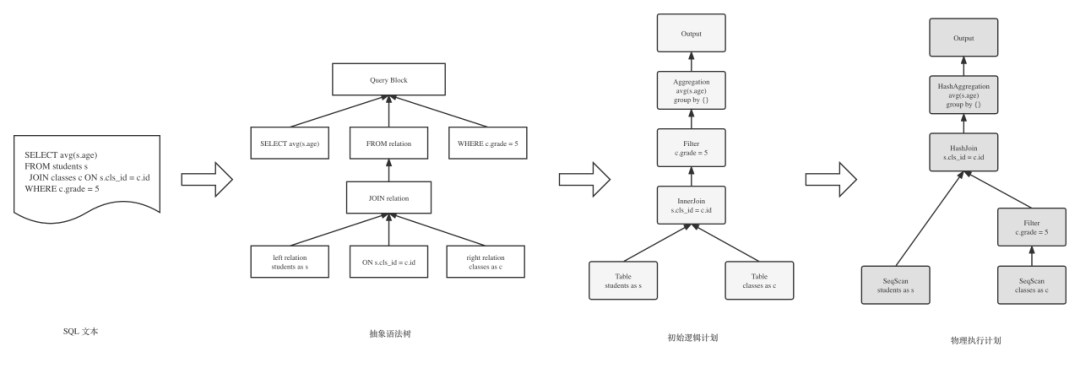The optimizer converts the abstract syntax tree into the initial logical plan. Generally, it performs basic checks during this process, such as table existence and permission sufficiency. The logical plan undergoes a series of equivalent rewrites and selects how each operator should be executed (the operator implementation may vary), resulting in an executable physical plan. At this point, the core work of the optimizer is complete. The most critical part of the optimizer is the process from logic to physics. Let's go into the details.

## 3. Basic Optimization Principles

### 3.1 Rule-Based Optimization

A large part of the work in the optimizer is based on rules (Rule-Based Optimizer or RBO). In short, I will rewrite SQL into whatever form I think is better. For example, the filter condition pushdown mentioned above is a rule.

There are many such optimizations, including pushdown, cutting, simplifying expressions, and disassociating associations. It is also recommended to use the information hidden in SQL for deeper optimization.

``````/* Query the average age of the students in the first class */
SELECT avg(s.age)
FROM students s
JOIN classes c ON s.cls_id = c.id
WHERE c.id = 1``````

In this query, in addition to pushing down the filter condition, we can generate a condition `s.cls_id = 1` for the students' table because through, the condition of JOIN, we know s.cls_id is equivalent to c.id. Some data can be filtered out earlier to reduce the work of JOIN. After the final rewrite, the SQL statement is listed below:

``````SELECT avg(s.age)
FROM (
SELECT age, cls_id
FROM students
WHERE cls_id = 1 /* The condition generated according to the equivalence relation */
) s
JOIN (
SELECT id
FROM classes
WHERE id = 1 /* Push down the filter condition */
) c
ON s.cls_id = c.id``````

The rewritten SQL is still not optimal because the data output by the two tables can join with the other side. Therefore, the number of lookup computations generated by INNER JOIN `s.cls_id = c.id` and columns that do not need to be output can be omitted. This rewriting is equivalent. If one side cannot query data, there will be no results after joining.

``````SELECT avg(s.age)
FROM (
SELECT age
FROM students
WHERE cls_id = 1
) s
JOIN (
SELECT NULL
FROM classes
WHERE id = 1
) c``````

This is some basic information about SQL optimization. However, there is a problem that is easily overlooked – which is on the left and which is on the right when these two tables are joined?

In general, you must apply HASH JOIN if you want to achieve INNER JOIN. Join the right-side data to build HashSets, and the left-side data is used to find HashSets. If you can find a HashSet, it means this line can match, and this line needs to be output. There is no doubt that you all will put the class table on the right because the classes table is smaller, thus making HashSet construction efficient, taking up less memory, and accelerating queries.

However, the optimizer does not know the business relationship between students and classes, nor does it know that there is an order of magnitude difference between students and classes in data volume. This is where cost-based optimization comes in handy.

### 3.2 Cost-Based Optimization

Most of the earnings of SQL optimization come from the optimization of the cost-based optimizer (CBO). As many effective optimization methods cannot be 100% sure if they have earnings, it is necessary to evaluate earnings by estimating the cost of execution. CBO is standard in the optimizer, and it is only a matter of depth of implementation between different products. Estimation is a core in CBO. This problem was thoroughly studied more than ten years ago and has mature applications in commercial databases. However, the estimation is not 100% accurate, which is a problem that has not been solved. Therefore, much research in optimizers is around inaccurate estimation, thus deriving many technical solutions.

Let's return to the example above. How does the optimizer know how many rows are left in each of the two tables after they are filtered? The concept of statistics needs to be introduced here. Statistics are the information collected by analyzing the data in the table in advance. Most databases support manually execute `ANALYZE` command to collect statistics. Commercial data warehouse products (such as AnalyticDB for MySQL) generally support automatic collection.

The statistics to be used in this example are the number of distinct values (NDV) and uniformity assumption. Assume there are 1000 students and five classes, and the students are evenly distributed in the classes.

Then,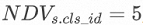,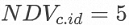. The estimation results are listed below:

• The estimated result of `s.cls_id = 1` is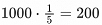.
• The estimated result of `c.id = 1` is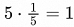.

Based on the estimation results, the optimizer puts students on the left and uses the classes table to build HashSets. It seems to be quite simple because this is a simple and typical relationship between the fact table and the dimension table, and each class size is similar, which is in line with the uniformity assumption model. If you change the condition to `s.cls_id >= 1`, you need to reintroduce the range statistics min & max. Let's make it more complicated. Assume the actual number is uneven, and some classes have no students. Therefore, the number of classes may exceed the number of students when you query in an interval. Then, the left and the right need to be exchanged. In order to deal with non-uniform models (most business data is not evenly distributed), you need histogram statistics to solve the problem. In addition, only a single filter condition is estimated here. In practice, you have to estimate combined conditions to estimate the JOIN results, significantly increasing the difficulty.

#### 3.2.1 Histogram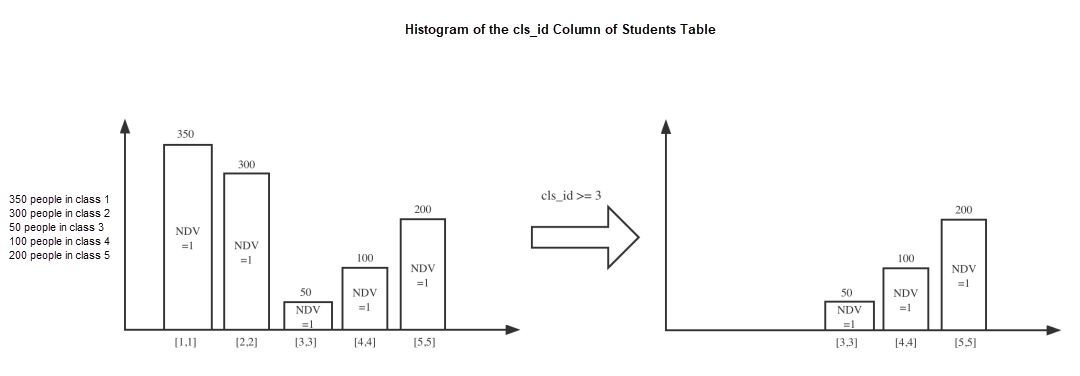Histogram is a necessary capability for commercial data warehouses. Some open-source data warehouse products have histograms, but they are not complete. For example, they can only estimate filters with histograms but not joins. Histograms are generally divided into equal width and equal height. The equal height is more frequently used because they can deal with extreme values better.

In the preceding figure, if you use only basic statistics (Min=1, Max=5, NDV=5), the estimated result is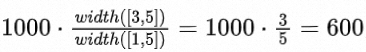, which is overestimated with an error of 71%. The estimated result is 350 with the histogram, which is accurate. This example is slightly special because each value is described by a bucket, so the estimated solution must be accurate.

In practice, it is impossible to create a bucket (precise histogram) for each value, and the number of buckets is generally limited to reduce histogram computation and storage overhead. At this time, multiple values will be divided into a bucket, with the method of equal width and height mentioned earlier. Such a histogram would be relatively blurred because the details of each value in each bucket are unclear. Generally, you can balance the estimation error and overhead with 100 to 300 buckets.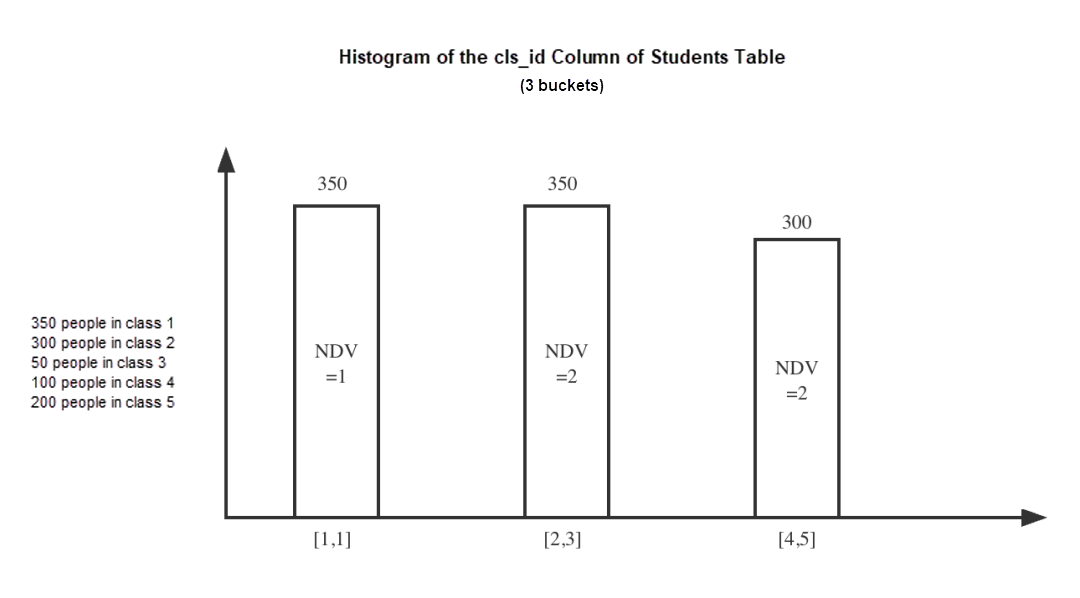In AnalyticDB for MySQL, the system automatically identifies and selects the histogram to be created. The establishment of an accurate histogram is more appropriate for the small NDV mentioned above. Even if an accurate histogram is not created, AnalyticDB for MySQL would identify some hot values and put them in a separate bucket to increase the estimation accuracy.

#### 3.2.2 Underestimation Error

The section above briefly introduced the way to estimate the number of rows, but in practice, you need to estimate the changes in the range, NDV, and other information, after each operator. This is handled by a module called Cardinality Evaluation (CE) in the optimizer. In addition, you need to estimate the cost (CPU/I /O/MEM/NET) of each operator based on the information provided by CE and Cost Model because the actual process is not as simple as determining whether to join the left or the right. The JOIN order may be factorial-level. Different orders have an impact on the amount of computation of each operator. Different operators have different overheads when processing the same number of rows. Therefore, the final optimizer needs to select the plan with the lowest total cost. Cost estimation is one of the more complex techniques in the optimizer.

It seems that choosing the least costly plan is the correct idea. With these complex operations, the best plan is sometimes not chosen because there are errors in the estimation, and most of the errors come from the CE module. The causes of the error are usually the following:

1. Insufficient Estimation Capabilities: For example, no support for histograms makes it difficult to effectively deal with uneven data.
2. The use of the approximate algorithm during the collection of the statistical information, which has errors and is out-of-date
3. Unknown relationship among columns, affecting the estimation results
4. Cumulative Errors: After each operator, the derived statistical information has errors, which are accumulated.
5. Expressions that are hard to evaluate, such as LIKE expressions without constant prefixes

Although there are errors, they do not necessarily affect plan selection. Otherwise, the theory would not be mature and applied. As far as the example above is concerned, the difference in the amount of data between the two tables is large. Even if the histogram is not introduced to make a relatively accurate estimation, their relative size is still stable within the error range.

In any case, the more accurate the estimation, the better. There are many solutions to the problems above. For example, AnalyticDB for MySQL automatically analyzes the complexity of SQL and determines whether to dynamically sample complex filters to improve plan quality. There are also some techniques (like Adaptive Query Processing) that automatically correct the plan in the running state. Even for operators like JOIN that are difficult to estimate, in addition to traditional techniques, the industry has some theories that can be used to improve estimation accuracy. For example, Wander Join (sampling approximation) is used to estimate the intermediate results of JOIN.

### 3.3 Search Framework

As mentioned above, you have to choose the lowest-cost plan. Here, it involves how to efficiently find all possible plans and choose the lowest cost. The most classic scheme is the System-R style, which is still used by many open-source databases. This scheme is mainly based on RBO and cost-based join reorder (bottom-up DP). It features simplicity and efficiency. OLTP databases (like MySQL) use this scheme. The disadvantage is that it is easy to fall into a local optimum rather than a global optimum. For example, changes in the join order may affect the amount of data in shuffle. These factors need to be searched together to find the global optimum.

Commercial databases/data warehouses and open-source products are search frameworks built on Cascades theory (such as the well-known SQLServer, Snowflake, GreenplumDB, and Calcite). Cascades is currently the most mature theory, and the CBO of AnalyticDB for MySQL is also a search framework based on this theory. Cascades has the following advantages over traditional frameworks:

• It changes from bottom-up search to top-down search, which has more cutting opportunities and is more efficient.
• Layered search (logical search and then physical search) to unified search (logical search and physical search are mixed), which can trigger cutting earlier and has high efficiency.
• After the framework highly abstracts the optimizer work, it can be searched in parallel and is more extensible.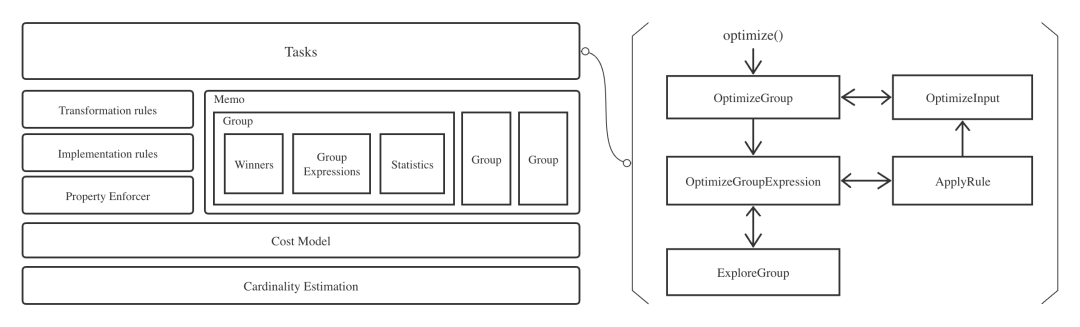The working principle of Cascades is tree-type dynamic programming (DP), which achieves top-down advance through memorization search. The working principle of each module is listed below:

#### 3.3.1 Expression

For example, JOIN and Filter are Expressions. The child nodes of an Expression are also Expressions, and together they form the plan tree. An Expression can be logical or physical.

#### 3.3.2 GroupExpression

GroupExpressions are similar to Expressions, except that the child nodes are Groups. This is more abstract. The purpose of abstraction is to reduce the number of expressions. If the child node has ten equivalent representations, there is no need to derive ten expression objects. Similarly, there are logical and physical GroupExpressions. If there is no special description below, the expressions generally refer to GroupExpressions.

#### 3.3.3 Group

A Group is a point in the plan tree. It is used to summarize repeated information to improve efficiency. It mainly includes:

1) Equivalent GroupExpression, logical, and physical. The resulting equivalent expressions are put into the same Group.

2) Some logic attributes of the Group: output statistics, cost lower limit, and output attribute information (unique, function dependency, ...)

3) The optimal solution (winner) for a specific request, which is the optimal result of a certain state in DP

#### 3.3.4 Winner

Winner stores the optimal solution for a particular request, which is a manifestation of a memory search. This request refers to the attribute requirements of the parent node on the child node, such as requiring the child node to give the optimal solution of distributing according to (A, B).

#### 3.3.5 PropertyEnforcer

If the child node cannot meet the requirements of the parent node, you need to insert an operator to meet the requirements. This is what the enforcer does. It decides what kind of operator to insert to meet the attribute requirements. Attributes mainly include distribution attributes and sorting attributes.

#### 3.3.6 Memo

Memo is the search space used to store Groups. After you generate a new expression, you need to go to Memo to find out whether the same or equivalent expression exists. If so, you need to put it into the same group.

#### 3.3.7 Rules

There are two categories:

1. Transformation Rules: It is responsible for transforming logical expressions into equivalent logical expressions. This step can generate new logical expressions.
2. Implementation Rules: It is responsible for converting logical expressions into physical expressions. This step can generate new physical expressions. For example, the implementation of JOIN can be Hash Join or Nested Loop Join.

These things are driven by various Tasks, which are essentially an abstraction of the process of recursively traversing the tree (recursion -> manual stack). This makes the search process more independent, scalable, and capable of parallel execution.

##### OptimizeGroup

OptimizeGroup initiates a specific request for specific Groups, which is the entry point for the optimization of each Group. In this step, all GroupExpressions in Groups are traversed. The logical expressions call OptimizeGroupExpression, and the physical expressions call OptimizeInput. OptimizeInput is run first to produce a materialized plan faster, so a cost upper bound is obtained to compress the subsequent search space.

##### OptimizeGroupExpression

OptimizeGroupExpression is to optimize specific logical expressions. The transformation and implementation rules are mainly applied. Call ApplyRule for each rule that can be applied. In particular, sometimes only transformation rules are applied, depending on the scenarios where OptimizeGroupExpression is called. Since different rules need to match different patterns, you may need to continue to explore sub-groups to know whether the pattern is met. So, before calling ApplyRule, if necessary, call ExploreGroup to explore sub-groups.

##### ExploreGroup

ExploreGroup is called by OptimizeGroupExpression. It is to explore all the equivalent logical expressions that may be generated within the Groups, preparing for ApplyRule. The discovery process is all about applying applicable transformation rules to all logical expressions in the Groups. This process reuses the code of OptimizeGroupExpression and only applies transformation rules. Therefore, it needs to call OptimizeGroupExpression.

##### ApplyRule

The input of ApplyRule is logical expressions and rules. Depending on the rule type, a new logical or physical expression is finally obtained. If a physical expression is generated, call OptimizeInput. If logic is generated, call OptimizeGroupExpression.

##### OptimizeInput

The input of OptimizeInput is a physical expression and property requirement. OptimizeInput generates a request for its subgroups based on physical expressions and attribute requirements and then initiates OptimizeGroup to all subgroups. The subgroups eventually return the winners that meet the requirements. According to the cost of these winners and the cost of the current physical expressions, the cost of the subtree rooted in the physical expressions is obtained. If this physical expression cannot meet the attribute requirements of the parent node in the end, PropertyEnforcer is required to insert a specific expression to meet the requirements.

#### 3.3.9 Branch Cutting

How does the cutting happen? When a request is sent to a subgroup, Winners are continuously collected. If the current accumulated cost has exceeded the upper bound, the search can be stopped directly. How does the upper bound be determined? In the beginning, it is infinite. As the first physical expression meets the requirements of the parent node, it will get a cost, which is regarded as the initial upper bound and brought to other tasks through the request context (different request contexts are separated, and upper bounds are independent). There may be many physical expressions in a group that can meet the requirements equivalently. Each physical expression will eventually be called OptimizeInput. In this process, some physical expressions can achieve a lower cost, so the upper bound in the context will be compressed. If some cannot meet the upper bound requirements, the search will stop directly. This is upper-bound cutting, which is used to reduce the search space and improve efficiency.

In addition to upper boundary cutting, there is lower boundary cutting. We mentioned earlier that the Optimizeinput is a physical expression, and we call OptimizeGroup for all subgroups. In this process, all subgroups may be explored, and the expression with the lowest cost is returned as Winner. If the cost exceeds the upper bound when a subgroup is explored, upper bound cutting will occur, and any subsequent search tasks will be directly stopped. Is it possible that you can directly know that the cost in the future will exceed the upper bound without exploring subgroups?

This is lower-bound cutting. You need to directly determine the minimum overhead (lower bound) of a Group based on the cost model and logical attributes. Any cost that may be calculated will be greater than or equal to this cost and recorded as `Group.costLowerBound`. If `sum(subGroup.costLowerBound) + GroupExpression.cost > upper bound` is workable, there is no need to explore subgroups. Stop directly.

These cutting techniques are effective and have been applied in AnalyticDB for MySQL. After our test, in TPC-DS, most of the query search space is reduced, and some can reduce the search space by nearly 50%.

## 4. Conclusion

Query optimization is important in related fields (such as databases and big data). The problems in actual production are far more complicated than what has been mentioned in this article. Since the article is short, we did not delve into more technical details.

AnalyticDB for MySQL is a new-generation cloud-native data warehouse that supports high concurrency and low latency queries. It is highly compatible with the MySQL protocol and SQL standards, allows multi-dimensional and real-time analysis and business exploration of large amounts of data, and supports the quick establishment of cloud-based data warehouses for enterprises.

0 1 0
Share on

# Related Products

• ##AnalyticDB for MySQL

AnalyticDB for MySQL is a real-time data warehousing service that can process petabytes of data with high concurrency and low latency.

• ##AnalyticDB for PostgreSQL

An online MPP warehousing service based on the Greenplum Database open source program

• ##ApsaraDB RDS for SQL Server

An on-demand database hosting service for SQL Server with automated monitoring, backup and disaster recovery capabilities